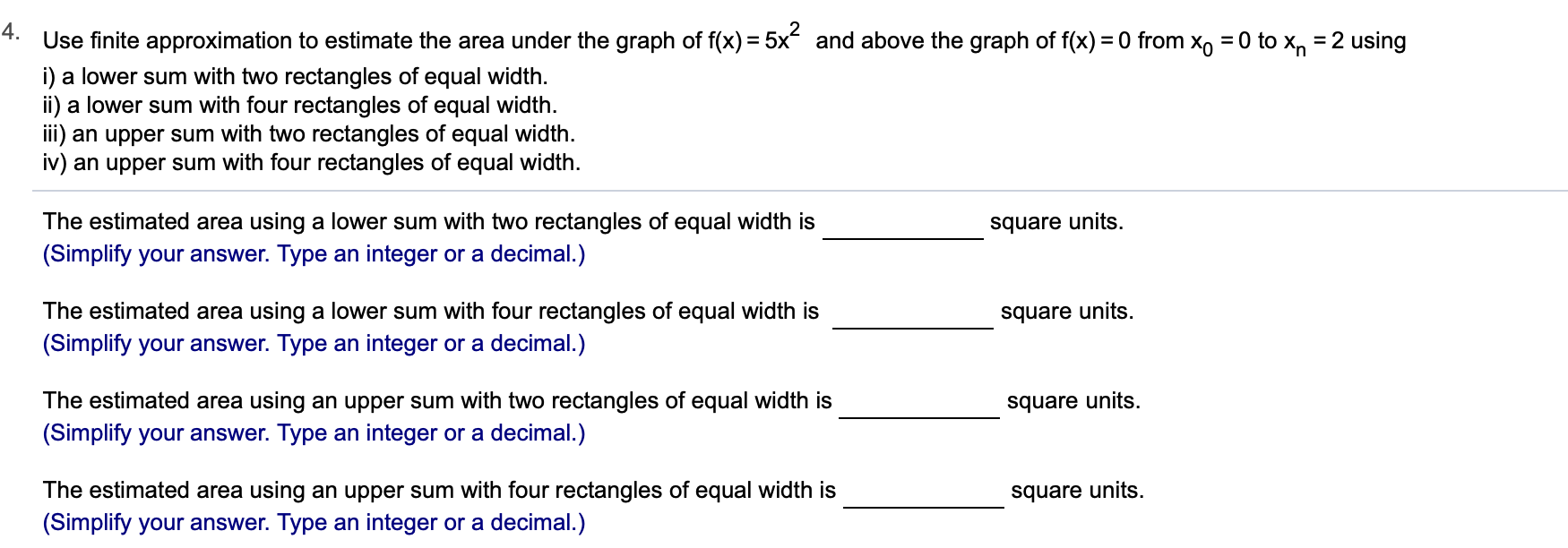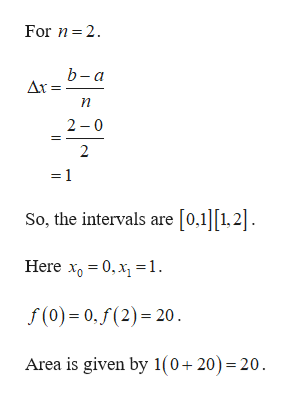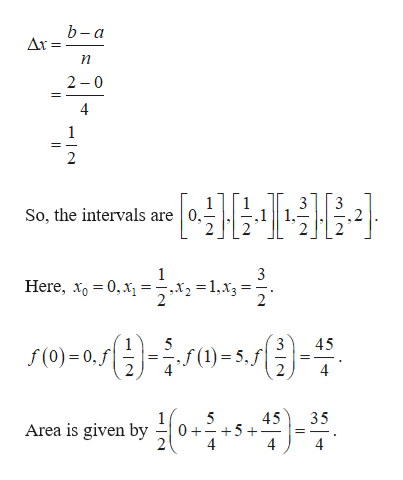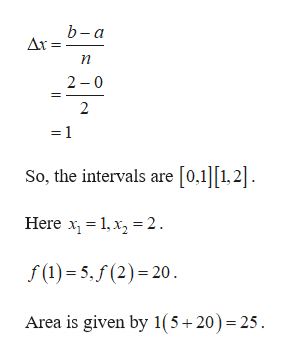# 4.Use finite approximation to estimate the area under the graph of f(x) 5xi) a lower sum with two rectangles of equal widthii) a lower sum with four rectangles of equal widthi) an upper sum with two rectangles of equal width.iv) an upper sum with four rectangles of equal widthand above the graph of f(x) 0 from Xo = 0 to x, = 2 usingThe estimated area using a lower sum with two rectangles of equal width is(Simplify your answer. Type an integer or a decimal.)square unitsThe estimated area using a lower sum with four rectangles of equal width issquare units(Simplify your answer. Type an integer or a decimal.)The estimated area using an upper sum with two rectangles of equal width issquare units(Simplify your answer. Type an integer or a decimal.)The estimated area using an upper sum with four rectangles of equal width is(Simplify your answer. Type an integer or a decimal.)square units

Question
74 views

Can i get help step by step with this problem pleasehelp_outlineImage Transcriptionclose4. Use finite approximation to estimate the area under the graph of f(x) 5x i) a lower sum with two rectangles of equal width ii) a lower sum with four rectangles of equal width i) an upper sum with two rectangles of equal width. iv) an upper sum with four rectangles of equal width and above the graph of f(x) 0 from Xo = 0 to x, = 2 using The estimated area using a lower sum with two rectangles of equal width is (Simplify your answer. Type an integer or a decimal.) square units The estimated area using a lower sum with four rectangles of equal width is square units (Simplify your answer. Type an integer or a decimal.) The estimated area using an upper sum with two rectangles of equal width is square units (Simplify your answer. Type an integer or a decimal.) The estimated area using an upper sum with four rectangles of equal width is (Simplify your answer. Type an integer or a decimal.) square units fullscreen
check_circle

Step 1

It is given that f(x) = 5x2, x0 = 0 and xn=2.

(i)help_outlineImage TranscriptioncloseFor n 2 b-а Ar = п 2 0 2 1 So, the intervals are [0,1][1,2] Here x0,x=1. f(o) 0,f(2)- 20 Area is given by 1(0+20)=20 fullscreen
Step 2

(ii)

For n=4help_outlineImage Transcriptioncloseb-a Ar = 2 0 So, the intervals are 0, 1 ,x2 = 1,xg Here, x0,x = 5 3 45 f(0) 0,f 2 35 5 0+ +5 4 45 Area is given by 4 fullscreen
Step 3

(iii)

For n=2

&nbs...help_outlineImage Transcriptioncloseb-a Δr- 2 0 2 =1 So, the intervals are [0.1]1,2 Here 1x2 f(1)5,(2) 20 Area is given by 1(5+20)= 25 fullscreen

### Want to see the full answer?

See Solution

#### Want to see this answer and more?

Solutions are written by subject experts who are available 24/7. Questions are typically answered within 1 hour.*

See Solution
*Response times may vary by subject and question.
Tagged in

### Calculus